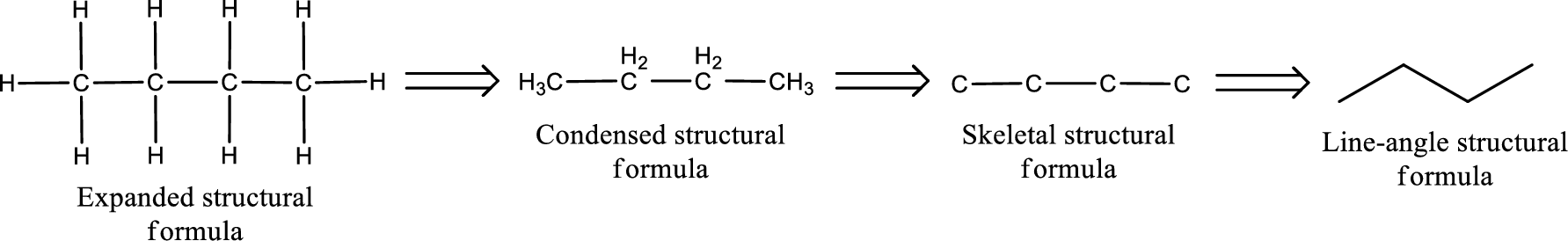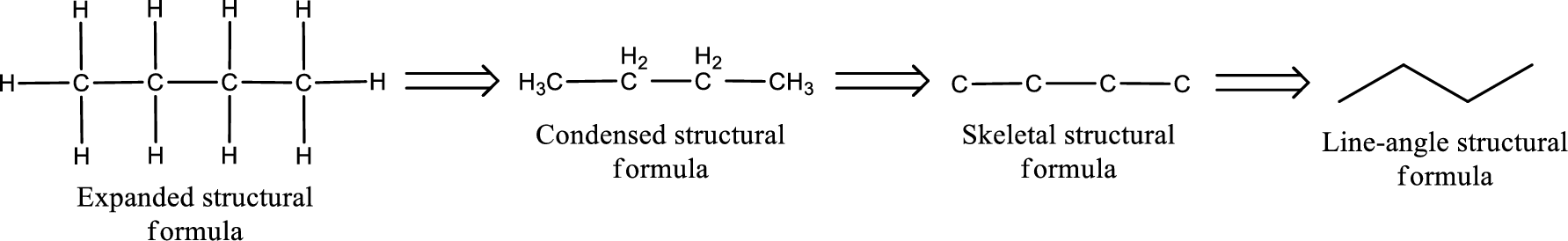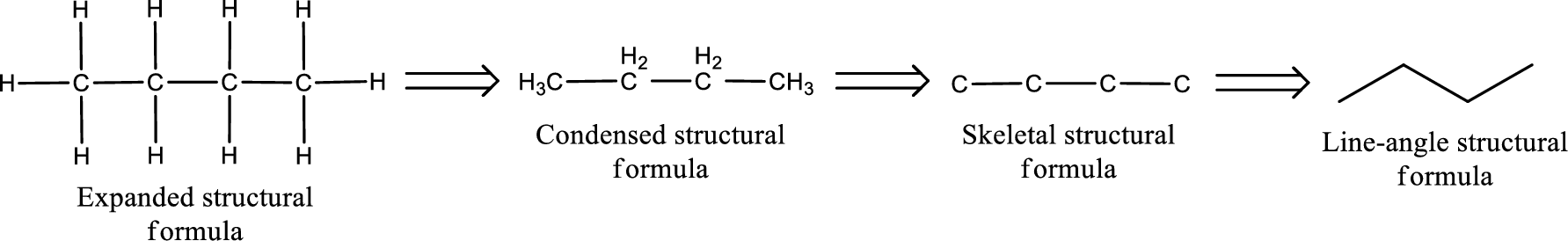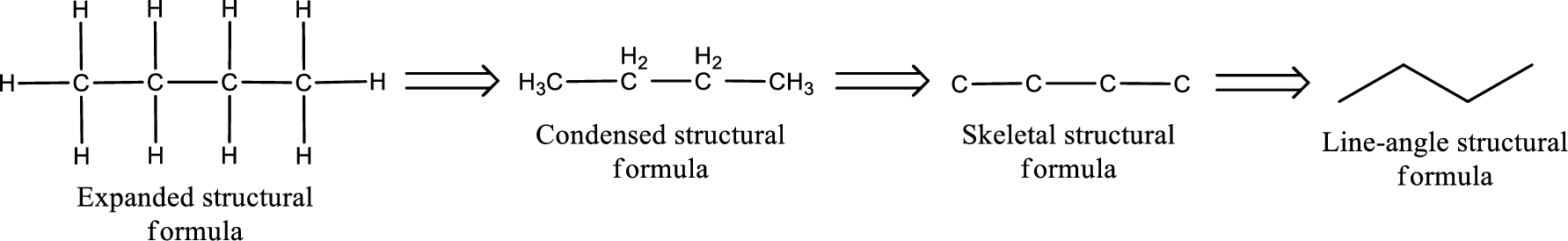Chapter 4, Problem 4.48EP### Organic And Biological Chemistry

7th Edition
STOKER + 1 other
ISBN: 9781305081079

#### Solutions

Chapter
Section### Organic And Biological Chemistry

7th Edition
STOKER + 1 other
ISBN: 9781305081079
Textbook Problem

# What is the molecular formula for each of the following compounds? a. Propanal b. Propanedial c. Propanone d. 2,4-Pentanedione

(a)

Interpretation Introduction

Interpretation:

Molecular formula for propanal has to be given.

Concept Introduction:

The structural representation of organic compound can be done in 2D and 3D.  In two-dimensional representation, there are four types of representation in which an organic compound can be drawn.  They are,

• • Expanded structural formula
• • Condensed structural formula
• • Skeletal structural formula
• • Line-angle structural formula

Structural formula which shows all the atoms in a molecule along with all the bonds that is connecting the atoms present in the molecule is known as Expanded structural formula.

Structural formula in which grouping of atoms are done and in which the central atoms along with the other atoms are connected to them are treated as group is known as Condensed structural formula.

Structural formula that shows the bonding between carbon atoms alone in the molecule ignoring the hydrogen atoms being shown explicitly is known as Skeletal structural formula.

Structural formula where a line represent carbon‑carbon bond and the carbon atom is considered to be present in each point and the end of lines is known as Line-angle structural formula.Molecular formula is the simple representation of different kind of atoms that are present in the compound.  This is represented as the number of atoms of same kind given in the subscript followed by the other element symbol.

Explanation

The given name of the compound is propanal.  From the name it is understood that the parent carbon chain is propane and it contains four carbon atoms.  The parent chain can be drawn as shown below,

From the name of the given compound, it is identified to be an aldehyde with no substituents present on the carbon chain.  The carbonyl carbon atom is the first carbon atom.

Carbon atom has a valence of four...

(b)

Interpretation Introduction

Interpretation:

Molecular formula for propanedial has to be given.

Concept Introduction:

The structural representation of organic compound can be done in 2D and 3D.  In two-dimensional representation, there are four types of representation in which an organic compound can be drawn.  They are,

• • Expanded structural formula
• • Condensed structural formula
• • Skeletal structural formula
• • Line-angle structural formula

Structural formula which shows all the atoms in a molecule along with all the bonds that is connecting the atoms present in the molecule is known as Expanded structural formula.

Structural formula in which grouping of atoms are done and in which the central atoms along with the other atoms are connected to them are treated as group is known as Condensed structural formula.

Structural formula that shows the bonding between carbon atoms alone in the molecule ignoring the hydrogen atoms being shown explicitly is known as Skeletal structural formula.

Structural formula where a line represent carbon‑carbon bond and the carbon atom is considered to be present in each point and the end of lines is known as Line-angle structural formula.Molecular formula is the simple representation of different kind of atoms that are present in the compound.  This is represented as the number of atoms of same kind given in the subscript followed by the other element symbol.

(c)

Interpretation Introduction

Interpretation:

Molecular formula for propanone has to be given.

Concept Introduction:

The structural representation of organic compound can be done in 2D and 3D.  In two-dimensional representation, there are four types of representation in which an organic compound can be drawn.  They are,

• • Expanded structural formula
• • Condensed structural formula
• • Skeletal structural formula
• • Line-angle structural formula

Structural formula which shows all the atoms in a molecule along with all the bonds that is connecting the atoms present in the molecule is known as Expanded structural formula.

Structural formula in which grouping of atoms are done and in which the central atoms along with the other atoms are connected to them are treated as group is known as Condensed structural formula.

Structural formula that shows the bonding between carbon atoms alone in the molecule ignoring the hydrogen atoms being shown explicitly is known as Skeletal structural formula.

Structural formula where a line represent carbon‑carbon bond and the carbon atom is considered to be present in each point and the end of lines is known as Line-angle structural formula.Molecular formula is the simple representation of different kind of atoms that are present in the compound.  This is represented as the number of atoms of same kind given in the subscript followed by the other element symbol.

(d)

Interpretation Introduction

Interpretation:

Molecular formula for 2,4-pentanedione has to be given.

Concept Introduction:

The structural representation of organic compound can be done in 2D and 3D.  In two-dimensional representation, there are four types of representation in which an organic compound can be drawn.  They are,

• • Expanded structural formula
• • Condensed structural formula
• • Skeletal structural formula
• • Line-angle structural formula

Structural formula which shows all the atoms in a molecule along with all the bonds that is connecting the atoms present in the molecule is known as Expanded structural formula.

Structural formula in which grouping of atoms are done and in which the central atoms along with the other atoms are connected to them are treated as group is known as Condensed structural formula.

Structural formula that shows the bonding between carbon atoms alone in the molecule ignoring the hydrogen atoms being shown explicitly is known as Skeletal structural formula.

Structural formula where a line represent carbon‑carbon bond and the carbon atom is considered to be present in each point and the end of lines is known as Line-angle structural formula.Molecular formula is the simple representation of different kind of atoms that are present in the compound.  This is represented as the number of atoms of same kind given in the subscript followed by the other element symbol.

### Still sussing out bartleby?

Check out a sample textbook solution.

See a sample solution

#### The Solution to Your Study Problems

Bartleby provides explanations to thousands of textbook problems written by our experts, many with advanced degrees!

Get Started

#### Find more solutions based on key concepts